Courses

# Test: Properties of Fluids & Turbulent Flow in Pipes - 1

## 20 Questions MCQ Test RRB JE for Mechanical Engineering | Test: Properties of Fluids & Turbulent Flow in Pipes - 1

Description
This mock test of Test: Properties of Fluids & Turbulent Flow in Pipes - 1 for Mechanical Engineering helps you for every Mechanical Engineering entrance exam. This contains 20 Multiple Choice Questions for Mechanical Engineering Test: Properties of Fluids & Turbulent Flow in Pipes - 1 (mcq) to study with solutions a complete question bank. The solved questions answers in this Test: Properties of Fluids & Turbulent Flow in Pipes - 1 quiz give you a good mix of easy questions and tough questions. Mechanical Engineering students definitely take this Test: Properties of Fluids & Turbulent Flow in Pipes - 1 exercise for a better result in the exam. You can find other Test: Properties of Fluids & Turbulent Flow in Pipes - 1 extra questions, long questions & short questions for Mechanical Engineering on EduRev as well by searching above.
QUESTION: 1

Solution:
QUESTION: 2

Solution:

Ans. (c)

QUESTION: 3

### The dimensions of surface tension is:

Solution:

Ans. (c) The property of the liquid surface film to exert a tension is called the surface tension. It is the force required to maintain unit length of the film in equilibrium. In Sl units surface tension is expressed in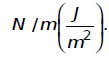In metric gravitational system of units it is expressed in kg(f)/cm or kg(f)/m.

QUESTION: 4

Assertion (A): In a fluid, the rate of deformation is far more important than the total deformation it self.
Reason (R): A fluid continues to deform so long as the external forces are applied.

Solution:

Ans. (a) This question is copied from Characteristics of fluid
1. It has no definite shape of its own, but conforms to the shape of the containing vessel.
2. Even a small amount of shear force exerted on a fluid will cause it to undergo a deformation which continues as long as
the force continues to be applied.
3. It is interesting to note that a solid suffers strain when subjected to shear forces whereas a fluid suffers Rate of Strain i.e. it flows under similar circumstances.

QUESTION: 5

What is the unit of dynamic viscosity of a fluid termed 'poise'equivalent to?

Solution:

Ans. (c)

QUESTION: 6

The shear stress in turbulent flow is:

Solution:

Ans. (b)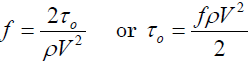QUESTION: 7

While water passes through a given pipe at mean velocity V the flow isfound to change from laminar to turbulent. If another fluid of specificgravity 0.8 and coefficient of viscosity 20% of that of water, is passed  through the same pipe, the transition of flow from laminar to turbulentis expected if the flow velocity is:

Solution:

Ans. (d) Rew =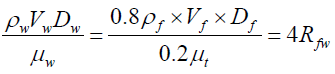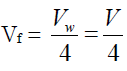QUESTION: 8

Flow takes place and Reynolds Number of 1500 in two different pipes with relative roughness of 0.001 and 0.002. The friction factor

Solution:

Ans. (c) The flow is laminar (friction factor,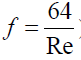) it is not depends on roughness but for turbulent flow it will be higher for higher relative roughness.

QUESTION: 9

The shear stress developed in lubricating oil, of viscosity 9.81poise, filled between two parallel plates 1 cm apart and moving with relative velocity of 2 m/s is:

Solution:

Ans. (b) du=2 m/s; dy= 1cm = 0.01 m;
μ = 9.81 poise = 0.981 Pa.s
Therefore (ζ) = μ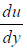= 0.981 ×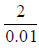= 196.2 N/m2

QUESTION: 10

An oil of specific gravity 0.9 has viscosity of 0.28 Strokes at 380C.What will be its viscosity in Ns/m2

Solution:

Ans. (c) Specific Gravity = 0.9 therefore Density = 0.9 × 1000 =900 kg/m3
One Stoke = 10-4 m2/s
Viscosity (μ) = ρν
= 900 × 0.28 × 10-4 = 0.0252 Ns/m2

QUESTION: 11

Assertion (A): In general, viscosity in liquids increases and ingases it decreases with rise in temperature.  Reason (R): Viscosity is caused by inter molecular forces of cohesion and due to transfer of molecular momentum between fluid layers; of which in liquids the former and in gases the later contribute the major part towards viscosity.

Solution:

Viscosity is higher in liquids than gases as the temperature increases. It will certainly change its state to gas and so the viscosity will decrease. The reason is true it is due to the friction between fluid layers.

QUESTION: 12

If the Relationship between the shear stress ζ and the rate of shear strain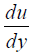is expressed as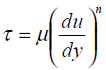then the fluid with exponent n>1 is known as which one of the following?

Solution:

Ans. (b)

QUESTION: 13

Match List-I with List-II and select the correct answer.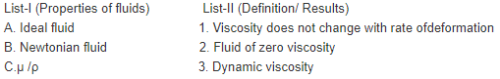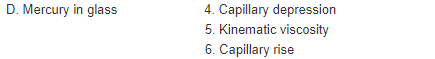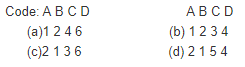Solution:

Ans. (d)

QUESTION: 14

What is the pressure difference between inside and outside of adroplet of water?
Where 'σ' is the surface tension and’d’ is the diameter of the droplet.

Solution:

Ans. (b) Pressure inside a water droplet,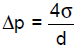QUESTION: 15

The pressure drop in a 100 mm diameter horizontal pipe is 50 kPa overa length of 10m. The shear stress at the pipe wall is:

Solution:

Ans. (b)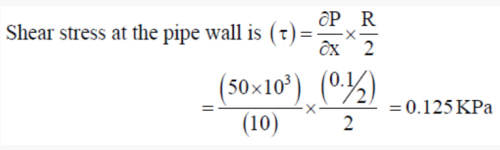QUESTION: 16

Reynolds stress may be defined as the

Solution:

Ans. (c) Reynolds stress may be defined as additional shear stresses due to fluctuating velocity components in a turbulent flow.

QUESTION: 17

As the transition from laminar to turbulent flow is induced a cross flowpast a circular cylinder, the value of the drag coefficient drops.

Solution:

Ans. (b) For turbulent flow total shear stress (ζ) =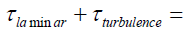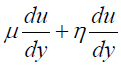QUESTION: 18

In turbulent flow over an impervious solid wall

Solution:

Ans. (d)

QUESTION: 19

If the surface tension of water-air interface is 0.073 N/m, thegauge pressure inside a rain drop of 1 mm diameter will be:

Solution:

Ans. (d) P =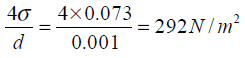QUESTION: 20

A capillary tube is inserted in mercury kept in an open container.Assertion (A): The mercury level inside the tube shall rise above the level of mercury outside.
Reason (R): The cohesive force between the molecules of mercury is greater than the adhesive force between mercury and glass.

Solution:

Ans. (d) Mercury shows capillary depression.